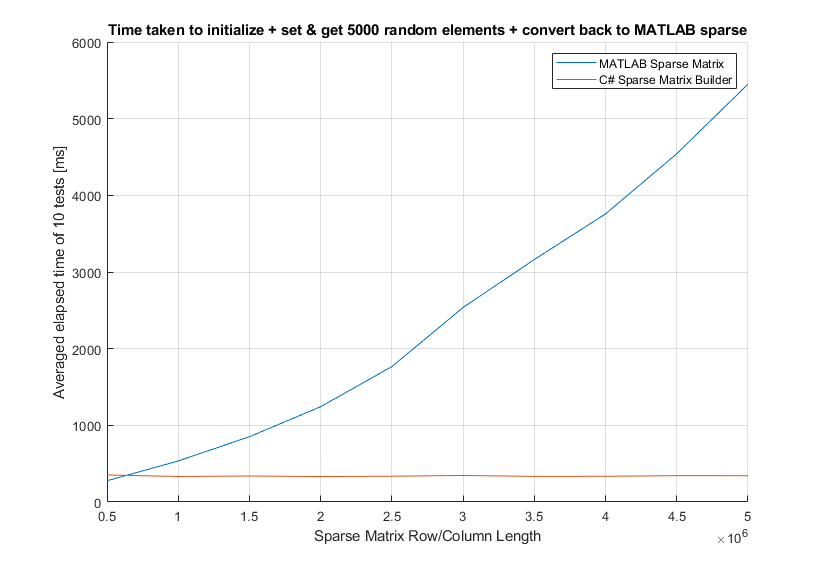## Fast Sparse Matrix Builder

バージョン 1.0.4 (29.2 KB) 作成者:
Much faster routines for creating and build huge sparse matrices in MATLAB. Latest version on github (file exchange seems to not autoupdate)

ダウンロード 24 件

GitHub から

# Sparse-Matrix-Builder

### By C. Souto (csouto@fe.up.pt)

Much faster routines for creating and building huge sparse matrices in MATLAB.

Usage example:

```% reset workspace
clear all; close all; clc;

% load the .NET assembly
dll = [pwd '\SparseLibrary.dll'];

% create a sparse matrix builder
m = 8;
n = 4;
matrix = SparseMatrixBuilder(m, n);

% set some values
matrix.Set(1, 3, 5.0);
matrix.Set(2, 4, 6.0);
matrix.Set(7, 1, 4.0);
matrix.Set(7, 3, 9.0);
%matrix.Set(9, 3, 1.0); % Error: Index was outside the bounds of the array.

% set multiple values
matrix.Set([1 2 3], [4 3 2], [10. 20. 30.]);

% add some values (same as set, except the value is added to the matrix element, while set replaces it)
%matrix.Set(9, 3, 1.0); % Error: Index was outside the bounds of the array.

% add multiple values (same as set, except the value is added to the matrix element, while set replaces it)
matrix.Add([1 2 3], [4 3 2], [10. 20. 30.]);

% get some values (print)
matrix.Get(7, 1)
matrix.Get(1, 1)
%matrix.Get(8, 5) % Error: Index was outside the bounds of the array.

% get multiple values
matrix.Get([1 2 3], [4 3 2])

% set all values in the diagonal
matrix.SetDiagonal(5.0)

% get the diagonal (print)
matrix.GetDiagonal()

% set and get a row
matrix.SetRow(2, 6.0);
matrix.GetRow(2) % will print

% set and get a column
matrix.SetColumn(2, 8.0);
matrix.GetColumn(2) % will print

% to dense (print)
matrix.ToDense()

% to COO format (print)
matrix.ToCOO()

% to MATLAB sparse (print)
matrix.ToMatlabSparse()```### 引用

Carlos Souto (2022). Fast Sparse Matrix Builder (https://github.com/carlosouto/Sparse-Matrix-Builder/releases/tag/v1.0.4), GitHub. 取得済み .

すべてのリリースと互換性あり
##### プラットフォームの互換性
Windows macOS Linux

### Community Treasure Hunt

Find the treasures in MATLAB Central and discover how the community can help you!

Start Hunting!

#### MATLAB/Tests

この GitHub アドオンでの問題を表示または報告するには、GitHub リポジトリにアクセスしてください。
この GitHub アドオンでの問題を表示または報告するには、GitHub リポジトリにアクセスしてください。